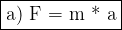## Which equation can correctly express the relation between force, acceleration, and mass? a) F = m × a b) F = m ÷ a c) F =

Question

Which equation can correctly express the relation between force, acceleration, and mass?
a) F = m × a
b) F = m ÷ a
c) F = m − a
d) F = m + a

in progress 0
6 months 2021-07-17T12:30:44+00:00 2 Answers 43 views 0

1. Hi there!According to Newton’s Second Law, force is calculated with the formula:

F = m × a, where:

F = force (N)

m = mass (kg)

a = acceleration (m/s²)